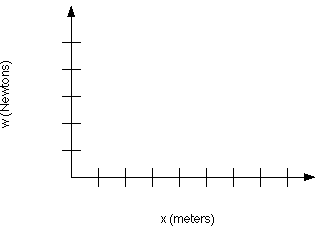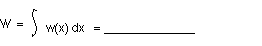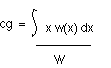PRINT THIS ANSWER SHEET TO FILL OUT AND TURN IN.

NAME_________________________________ CLASS____________________ DATE____________

### Determining Center Of Gravity (Level 2) Worksheet

1. What is the length of a Boeing 747-400 domestic airplane?

Length = __________

2. Let us suppose that the approximate weight distribution (function) on a loaded 747-400 domestic airplane is given by the equation,

w(x) = 700 sin(2px / L) + 42,850,

where x is the distance measured from the nose of the airplane. Graph the weight distribution.3. What is the total weight W of the airplane?4. Use the equation,to calculate the center of gravity of the airplane. The integration is calculated for x = 0 (nose) to Length (tail).

[ Hint: Use integration by parts.]

cg = __________________

5. Let us suppose that the approximate weight distribution (function) on a loaded 747-400 domestic airplane is given by the equation,
w(x) = 777 ( cos(2px / L) + sin(2px / L) ) + 42,850,

where x is the distance measured from the nose of the airplane. Graph the weight distribution.6. What is the total weight W of the airplane?7. How does the weight calculated in Problem 3 compare with the weight in Problem 6? Explain why this is so.

Use the equation,to calculate the center of gravity of the airplane with the new weight distribution. The integration is calculated for x = 0 (nose) to Length (tail). [ Hint: Use integration by parts.]

cg = _____________

8. Did the center of gravity move?
1. If so, did it move toward the airplane's nose or tail?

2. If not, why did it stay in the same position?

9. If the airplane's center of gravity moved significantly during flight, do you think the pilot would notice it? Why or why not?